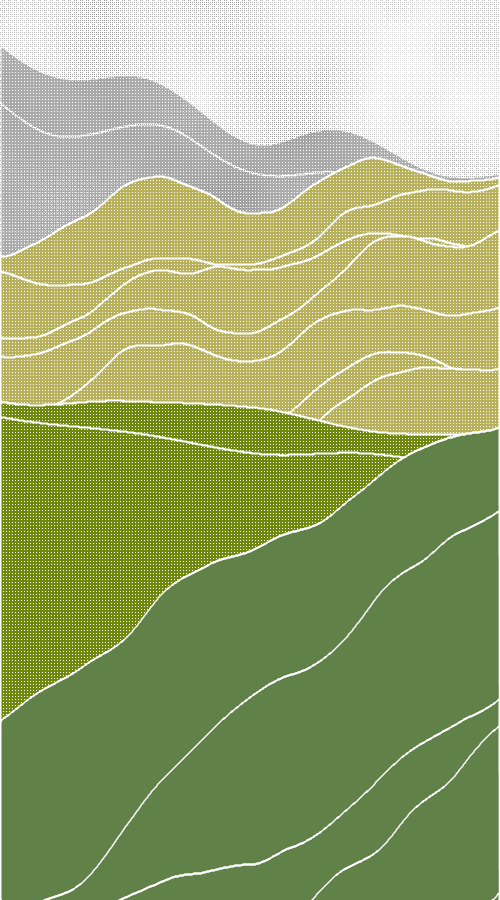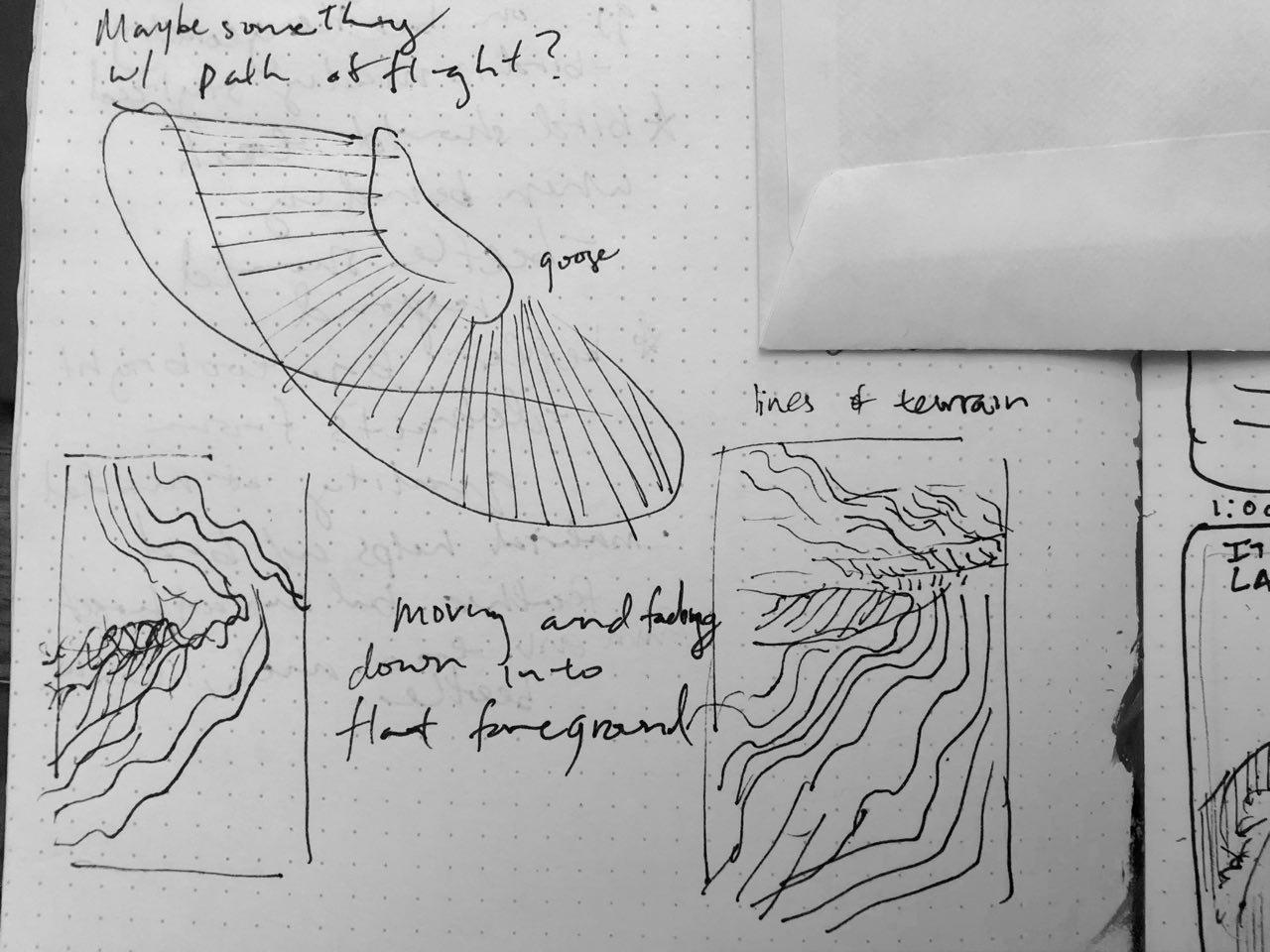# Jackalope-AnimatedLoop.My initial idea was to do something that was like hills, how they ebb and flow into one another in beautiful landscapes. That is one of the things I feel I have succeeded in so I’m happy with that. However, there are many problems I still have with this piece. I’ve never used Processing before and I feel like that considerably slowed my making of this. There is a lot that I didn’t realize was possible with Processing until midway into this project and a lot that I didn’t think I’d be able to do, and so I also feel like the gif I made is a bit simplistic. The easing function I used was a Double-Circular Ogee, which I chose because I liked how it caused the sun and moon to slow as they neared the the end of their cycles. I also liked the lazier, more serene drift of the moon across the sky compared to the sun.```//Special thanks to Golan Levin for providing code to generate frames and this blog //about using noise https://necessarydisorder.wordpress.com/2017/11/15/drawing-from-noise-and-then-making-animated-loopy-gifs-from-there/   // This is a template for creating a looping animation in Processing/Java. // When you press the 'F' key, this program will export a series of images // into a "frames" directory located in its sketch folder. // These can then be combined into an animated gif. // Known to work with Processing 3.3.6 // Prof. Golan Levin, January 2018   //=================================================== // Global variables. String myNickname = "Jackalope"; int nFramesInLoop = 120; int nElapsedFrames; boolean bRecording;   //=================================================== void setup() { size (500, 900); bRecording = false; nElapsedFrames = 0; } int start = 300; float function_PennerEaseInOutCubic (float x) { String functionName = "Penner's EaseInOut Cubic";   x *= 2.0; float y = 0;   if (x < 1) { y = 0.5 * x*x*x; } else { x -= 2.0; y = 0.5 * (x*x*x + 2.0); } return y; }   //=================================================== void keyPressed() { if ((key == 'f') || (key == 'F')) { bRecording = true; nElapsedFrames = 0; } }   //=================================================== void draw() {   // Compute a percentage (0...1) representing where we are in the loop. float percentCompleteFraction = 0; if (bRecording) { percentCompleteFraction = (float) nElapsedFrames / (float)nFramesInLoop; } else { percentCompleteFraction = (float) (frameCount % nFramesInLoop) / (float)nFramesInLoop; }   // Render the design, based on that percentage. renderMyDesign (percentCompleteFraction);   // If we're recording the output, save the frame to a file. if (bRecording) { saveFrame("frames/" + myNickname + "_frame_" + nf(nElapsedFrames, 4) + ".png"); nElapsedFrames++; if (nElapsedFrames >= nFramesInLoop) { bRecording = false; } } }   //=================================================== void renderMyDesign (float percent) { // // YOUR ART GOES HERE. // This is an example of a function that renders a temporally looping design. // It takes a "percent", between 0 and 1, indicating where we are in the loop. // This example uses two different graphical techniques. // Use or delete whatever you prefer from this example. // Remember to SKETCH FIRST!   //---------------------- // here, I set the background and some other graphical properties background (138, 165, 30); smooth(); stroke(255); strokeWeight (2); float damping; float t = map(percent, 0, 1, 0, TWO_PI); float px = width/2.0 + .05* cos(t); float py = width/2.0 + .05* sin(t);   loadPixels(); for (int x = 0; x<width; x+=1) { for (int y = 0; y<height; y+=1) { float ns = (float)noise(.01*x, .01*y, px*100); float col = map(ns, -1, 1, 0, 100); pixels[x + width*y] = color(255, col); } } updatePixels(); //moon and sun float easeP = function_DoubleCircularOgee(.07, percent); if (percent<.5) background((1-percent)*255); else background(percent*255); fill(222, 95, 20); float cx = width*.5; float cy = height*.25; float sx = cx+250*cos(2.5*TWO_PI*easeP); float sy = cy-150*sin(2.5*TWO_PI*easeP); ellipse(sx, sy, 50, 50);   fill(255); float mx = cx-250*cos(2.5*TWO_PI*easeP); float my = cy+150*sin(2.5*TWO_PI*easeP); ellipse(mx, my, 50, 50);       //some sin waves   int lines=10; fill(184, 176, 90); pushMatrix(); shearY(PI/10.0); for (int line = start; line<height*.5; line+=(height/lines)) { beginShape(); vertex(0, height); for (int i = 0; i<width; i++) { float x = i; float y = line/2-height/5+160*noise(px+line+line*.003, py+line+i*.002)+15*sin(i*.01*PI); if (x>=0 &&x<=width) vertex(x, y); } vertex(width, height*2); endShape(CLOSE); } popMatrix(); loadPixels(); for (int x = 0; x<width; x+=2) { for (int y = 0; y<height; y+=2) { float ns = (float)noise(.01*x, .01*y, px*100); float col = map(ns, -1, 1, 0, 255); pixels[x + width*y] = color(col); } } updatePixels(); px = width/2.0 + .09 * cos(t); py = width/2.0 + .09 * sin(t); pushMatrix(); shearY(-PI/15.0); for (int line = 0; line<height*.7; line+=(height/lines)) { beginShape(); vertex(0, height); for (int i = 0; i<width; i++) { float x = i; float y = line*.4+height*.2+160*noise(px+1.5*line+line*.003, py+line+i*.004)+15*sin(px*20+i*.009*PI); if (x>=0 &&x<=width) vertex(x, y); } vertex(width, height*2); endShape(CLOSE); } popMatrix();   fill(110, 137, 14); loadPixels(); for (int x = 0; x<width; x+=3) { for (int y = 0; y<height; y+=3) { float ns = (float)noise(.01*x, .01*y, px*100); float col = map(ns, -1, 1, 0, 255); pixels[x + width*y] = color(col); } } updatePixels(); px = width/2.0 + .1 * cos(t); py = width/2.0 + .1 * sin(t); pushMatrix(); shearY(PI/20.0); for (int line = 0; line<height; line+=(height/2)) { beginShape(); vertex(0, height); for (int i = 0; i<width; i++) { float x = i; float y = line*.09+height*.3+300*noise(px+5.5*line+line*.0001, py+ line+i*.001)+10*sin(px*10+i*.001*PI); if (x>=0 &&x<=width) vertex(x, y); } vertex(width, height*2); endShape(CLOSE); } popMatrix();   loadPixels(); for (int x = 0; x<width; x+=3) { for (int y = 0; y<height; y+=3) { float ns = (float)noise(.01*x, .01*y, px*100); float col = map(ns, -1, 1, 0, 255); pixels[x + width*y] = color(255, col); } } updatePixels();     fill(96, 129, 71); px = width/2.0 + .2 * cos(t); py = width/2.0 + .1 * sin(t); pushMatrix(); shearY(-PI/5.0); for (int line = 0; line<height; line+=(height/8)) { beginShape(); vertex(0, height); for (int i = 0; i<width; i++) { damping = .0001*sq(i); float x = i; float y = line+height*.7+300*noise(px+5.5*line+line*.003, py+line+i*.003)+((30-damping)/100)*(15+1.1*damping)*sin(px*40+i*.01*PI); if (x>=0 &&x<=width) vertex(x, y); } vertex(width, height*2); endShape(CLOSE); } popMatrix(); }       //=================================================== // Taken from https://github.com/golanlevin/Pattern_Master   float function_DoubleCircularOgee (float x, float a){ // functionName = "Double-Circular Ogee";   float min_param_a = 0.0; float max_param_a = 1.0;   a = constrain(a, min_param_a, max_param_a); float y = 0; if (x<=a){ y = sqrt(sq(a) - sq(x-a)); } else { y = 1 - sqrt(sq(1-a) - sq(x-a)); } return y; }```